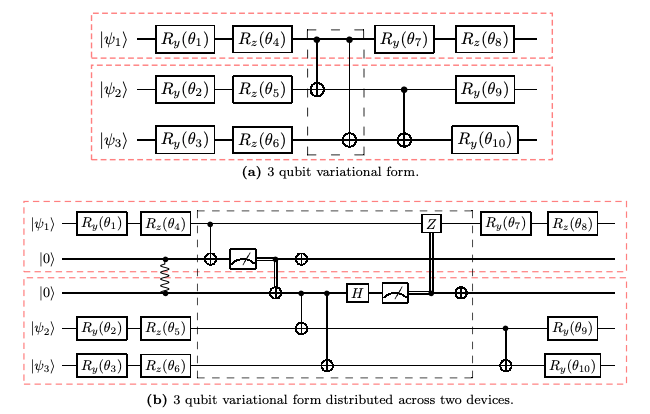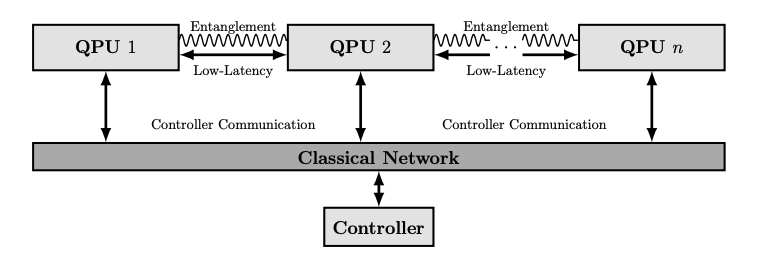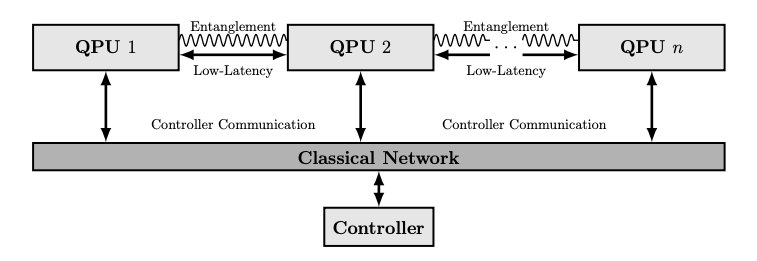## Interlin-q for distributed quantum computing: A path to large scale quantum computing

17 September, 2021

This post was originally published on Medium, and is being syndicated here.

A major obstacle for quantum computers to overcome in order to be useful for industrial scale problems is the need to be scaled up. Scaling quantum computers up to levels beyond the NISQ (i.e. 10s-100s of noisy qubits) era will require scientific breakthroughs and overcoming many current technological hurdles. One proposed solution to overcoming the scaling problem is to connect many smaller scale quantum processors together to form a distributed quantum computing. In this article I’ll summarize what a “distributed quantum computer” means and also discuss the challenges introduced when networking quantum computers. At the end, I will summarize and demo the Interlin-q project which aims to aid in studying some of the problems faced when dealing with distributed quantum computing.

To start off, it’s important to know what is meant by “scaling” when it comes to quantum computing. Scaling with regards to quantum computing generally refers to a few key things. Firstly the number of logical qubits that the quantum computer contains. “Logical” here effectively means a qubit that one can perform logical operations on. The number of logical qubits is important because as the size of the inputs — longer lists, bigger numbers, etc.— to a quantum algorithm grows, generally the number of qubits required to the perform the algorithm increases, and usually quite rapidly. For example, using Shor’s factoring algorithm, it takes 5 qubits to factor the number 15, but at the scale of 2048 bit RSA, the main cryptographic protocol for the Internet, it may require tens of millions of qubits (albeit not all logical). The current available quantum computers have in the range of hundreds of qubits, and it projected to take decades to reach millions of qubits with the current trends, although this is generally a controversial topic.

Another component to scaling up is the number of sequential operations (or logical gates) that can be applied to the qubits before too much noise is introduced to get any useable information out. This is generally referred to as the maximum circuit depth of the quantum computer. Circuit depth depends on a number of things, but most important are 1) the quality of technology performing the operations on the qubit, for example microwave or laser pulses, and 2) the quality of the qubits themselves, generally measured by how long they can remain in their quantum states before decohering to a classical state. These two properties are the main factors that govern how deep a circuit can be. Circuit depth is important because non-trivial quantum algorithms not only require many qubits, but will generally require a high circuit depth, and so being able to perform many sequential logical operations on the qubits will be of top importance for scaling.

Lastly, the next most common feature referred to when discussing scaling is how “connected” the quantum computer is, or “qubit connectivy”. What connectivity measures is effectively the number of qubits that each qubit can interact directly with. For example, if a quantum computer has n qubits and each qubit can interact directly with each other qubit, then the connectivity of this quantum computer is n-1. If on the other hand a qubit can only interact directly with one other qubit, then the connectivity is 1. This is important because when performing controlled operations (e.g. a CNOT gate), generally the qubits need to be physically near each other. If the qubits are not connected nor near, then moving the qubits to a place where such a controlled operation can be performed is required. This is usually done via what is known as a “swap chain” or via a teleportation protocol. The more connected a quantum computer is, the easier is it to perform controlled operations between arbitrary qubits, which has many implications when performing quantum algorithms in practice — a major one being the simplification of circuit compilation. Low connectivity implies a high complexity for circuit compilation which generally means longer runtimes and less time for performing logical operations. Increasing connectivity is a complex hardware problem and much work is also being done for improving algorithms for qubit routing within quantum computers.

The scaling problem is one of a fine balance between improving certain aspects while not affecting the others. For example, simply increasing the number of qubits in a quantum computer can make the connectivity more challenging. Or increasing the connectivity can make increasing circuit depth harder. To get a quick overall estimate for the quality of a quantum computer, the number of qubits, the maximum circuit depth, and the level of connectivity in a quantum computer have been combined to have a single measure called the quantum volume. Personally I think quantum volume is not a completely fair measure given the vast differences in technologies between the various quantum computer implementations (e.g. superconducting vs. trapped ion), so I usually refer to papers using the quantum computers to perform a quantum algorithm to get an estimate of their quality. Overall, the scale required to perform non-trivial quantum algorithms very rapidly increases with the problem complexity and so much research is being dedicated to methods of scaling up quantum computers.

One of the approaches to scaling that I find very interesting is distributed quantum computing. As it was done with classical computing when scaling became an issue, the concept of networking smaller processors together in order to distributing a computational load was introduced. For quantum computing, the same idea applies: Scaling quantum computers up is an issue, and so one can consider networking smaller quantum computers together. Naturally, since quantum computing varies drastically from classical computing, designing networked quantum computers introduces challenges that do not exist in purely classical networks. To more clearly understand these challenges, it helps to first understand what exactly a distributed quantum computing architecture could be.

A distributed quantum computer is a collection of quantum computers, nodes, where each quantum computer in the collection has a number of qubits on which it can operate. The quantum computers are connected via a network with which they can transmit classical- and, more importantly, quantum bits of information between themselves. A key difference for distributed quantum computing is that a connection to other nodes via a classical network alone is not enough to perform quantum computing in general, and so there must also be connection via a quantum network as well. A quantum network is the solution to the first major challenge for distributed quantum computing: Performing multi-qubit controlled operations (e.g. a CNOT gate) between qubits that are not physically located on the same quantum computer.

Because multiple qubits can be correlated via quantum entanglement, performing controlled operations becomes even more challenging across distributed quantum computers. Naturally due to the properties of quantum systems, one cannot simply measure the control qubit and transmit the classical information to the other computer. Rather, one needs to introduce another method which doesn’t measure the control qubit but yet still transmits the control information. This is usually referred to as a “non-local control operation” and are a number of ways to achieve this, one being quantum teleportation. Each method has resource requirement trade-offs and other than directly transmitting the control qubit, each of the methods has something in common: To transmit control information, an entanglement resource must first be created. Recently an experiment performing a non-local CNOT gate using a flying qubit (i.e. a single photon) entangled with the control qubit to transmit the control information was performed. When the flying qubit arrives at the other quantum computer, it interacts with the target qubit of the CNOT gate, completing the first part of the CNOT gate. To completely perform a CNOT gate, one needs to be aware of the “phase kick-back” that results from the states potentially being entangled. The last step of the non-local CNOT protocol is to extract the phase information from the flying qubit which becomes entangled with the target qubit after interaction. Luckily, this can be done by measuring the flying qubit and transmitting the single bit. Using the bit as control, a phase flip on the control qubit is performed or not.

The next major problem to overcome is that to perform distributed quantum computing, one has to remap quantum circuits designed for monolithic quantum computers (i.e. one single quantum computer) to a distributed architecture, filling in any missing pieces like the non-local control operations with equivalent steps. This requires two things: 1) Remapping monolithic circuits to distributed circuits; and 2) Preparing a precise instruction-execution schedule so that the two quantum computers work properly together. This introduces yet another step into the already non-trivial problem of quantum circuit compilation. These two problems are addressed in depth in a recent article I’ve co-authored with Marco Ghibaudi and James Cruise, but I will briefly review the approach we took for remapping and scheduling.In the above image, what we see is an example of the remapping from a monolithic circuit in (a) to an equivalent distributed circuit in (b). Here the red boxes indicate two networked quantum computers. This approach is not the only approach that can be used, and indeed there are others proposed, but for now we will stick with it since I know it best. The approach can be summarized as follows: Given an already optimized circuit designed for a monolithic quantum computer and the qubit topology of the distributed system, find any control gates that have control and target qubits not on the same quantum computer, and replace the local gate with its non-local equivalent — essentially a “find an replace”. There are some minor optimizations that I don’t describe, but that is the heart of it. One then feeds this distributed algorithm to a circuit scheduler.

To execute a distributed quantum algorithm, a precise execution schedule is required. In our article, we assume two different distributed topologies, the more straightforward in the below image. What the image shows is a network of quantum computers connected via a low-latency classical network, an entanglement network, and lastly an additional network which connects all of the quantum computers to a single controller. Such an architecture is common in mainframes for example, where a controller node dictates the programs to the computers in the cluster. Indeed we propose a similar idea. The single controller receives a circuit designed for the collection of quantum processing units (QPUs), or quantum computers, but designed assuming the n QPUs form monolithic system. The controller, knowing the distributed QPU topology, can perform the required remapping of the circuit to the distributed circuit. The next step, as already mentioned, is generating an execution schedule.The execution schedule uses a straight-forward approach using the assumption that the QPUs are all time-synchronized. When constructing the the distributed quantum computing, the timing specifications for the hardware components such as the latency of the cable, the timing of the gates, the entanglement generation rate, etc. will all be known. Given the timing parameters, the scheduling algorithm simply lines up the executions in time to then instruct the quantum computers to perform any distributed operation synchronously. The output of the scheduler is a list of hardware commands with a timestamp which is then distributed to the cluster of QPUs to begin the execution. Once complete, all measurement results are sent back to the controller, processed, and sent back to the user as if the algorithm was executed on a single QPU.In the article we analyze such a system and propose how this can be constructed as well as controlled using the Deltaflow.OS. What was left open from the work was a prototype of the proposed solution in simulation. In the next part of this post, I’ll discuss how we took the above ideas and translated them into a working simulation prototype.

Through the last two iterations of the Quantum Open Source Foundation’s mentorship program, Rhea Parekh, Ahmed Darwish, and I spent time working on developing a simulation platform for distributed quantum computing. The proposed solutions for distributing quantum circuits and scheduling from the work mentioned above were implemented and then simulating with parallel computation. We’ve dubbed this project “Interlin-q” and have now received supported by the Unitary Fund via their mirco-grant program.

Overall the plans for this project are to incorporate the various proposals and methods for performing distributed quantum computing into a simulator to show proof of concept. We moreover aim to study potential speedups that can be gained when taking particular quantum algorithms and putting them into a distributed and parallel setting. This beginning of this, we added in our arXiv write up of the current progress: Quantum Algorithms and Simulation for Parallel and Distributed Quantum Computing

Interlin-q is built on top of another framework called QuNetSim. QuNetSim is a quantum network simulator that simulates quantum network protocols up to the network layer. For more information about QuNetSim, there is a write up and a few YouTube videos as well. What Interlin-q does is it extends the network hosts of QuNetSim into two new objects, one which represents the controller of the overall system, and one that behaves purely as a controlled entity. These two objects behave like the components of network configuration of the image above.

The way a user interacts with Interlin-q is, they are expected to firstly define their distributed quantum computing architecture by setting the number of computing nodes in the system as well as how many qubits are on each node. One does this using the features of Interlin-q for building the distributed system. Next, the user programs their quantum circuit assuming all of the qubits in the distributed system exist on a single machine. To then start the simulation, the circuit is given to the controller node which performs the distribution mapping and creates a runtime schedule. The runtime schedule is distributed to the computing nodes and the simulation begins.

During the simulation, based on the execution schedule generated, the QuNetSim backend is triggered to send messages and generate entanglement between the computing nodes, as well as perform the logic gates in a synchronized fashion. At the end of the simulation, the computing nodes send all their measurement results back to the controller for processing and the user can then program what to do with the measurement results.

As a first non-trivial example of using Interlin-q, in the latest iteration of the mentorship program we implemented a parallized version of VQE much like the proposed approach in the Pennylane blog. One can review the Jupyter notebook of the example here. In a future post, I will go into detail of how this was implemented and the theory behind it.

Overall, we aim to continue our work with Interlin-q — tightening the screws, generating more examples, and implementing more examples from the literature. We hope to build up more interest in distributed quantum computing grow the community around it. The path to large scale quantum computing will inevitably make use of networked quantum computers and with that opens the door for many interesting questions!